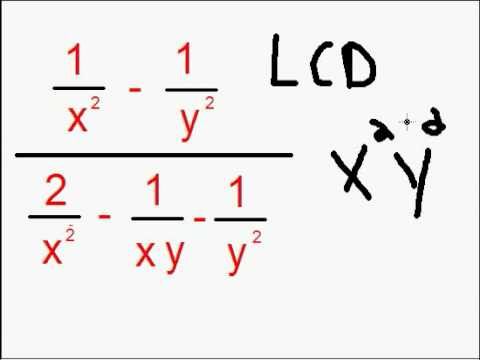## Simplification of Rational Expressions

Subject: Compulsory Maths

#### Overview

This note includes the information about the multiplication and division of Rational Expressions.

#### Simplification of Rational Expression

As we know that 1, 2, $\frac{1}{2}$,$\frac{-4}{5}$, etc are the rational numbers. Similarly,$\frac{x}{2}$,$\frac{x^2}{y^2}$,$\frac{x+3}{x-8}$, etc. are called rational expressions. Here we shall discuss about addition, subtraction,multiplication and division of rational expressions.

Multiplication of Rational Expressions

In multiplication, we simplify the numerical coefficients as in a case of multiplication of fraction. In the case of variables, we apply the product and quotient rules of indices. In a division, we should multiply the dividend by the reciprocal of a division. For example,

$\frac{4a^3b^2}{5a^3y^3}$×$\frac{10x^4y^3}{12a^4b}$

$\frac{2x^{4-3}× y^{3-2}}{3a^{4-3}×b^{3-2}}$

$\frac{2xy}{3ab}$ans.

Addition and Subtraction of Rational Expression with the different denominators

In this case, we should find the LCM of the denominators. Then the LCM is divided by each denominator and the quotient is multiplied by the corresponding numerator as in a case of simplification of unlike fraction. For example,

$\frac{x}{2}$ +$\frac{x}{6}$

$\frac{3x+x}{6}$ =$\frac{4x}{6}$ =$\frac{2x}{3}$ ans.

##### Things to remember
•  In a division, we should multiply the dividend by the reciprocal of a division.
• LCM is divided by each denominator and the quotient is multiplied by the corresponding numerator as in a case of simplification of unlike fraction.
• It includes every relationship which established among the people.
• There can be more than one community in a society. Community smaller than society.
• It is a network of social relationships which cannot see or touched.
• common interests and common objectives are not necessary for society.
##### Videos for Simplification of Rational Expressions##### Simplify Complex Rational Expressions

Solution:

$\frac{4a^3b^2}{5x^3y^2}$ × $\frac{10x^4y^3}{12a^4b^3}$

= $\frac{4a^3b^2×10x^4y^3}{5x^3y^2×12a^4b^3}$

= $\frac{2x^{4-3}×y^{3-2}}{3a^{4-3}b^{3-2}}$

= $\frac{2xy}{3ab}$

Solution:

= $\frac{5x^2}{8ax^3}$ ÷ $\frac{15a^3y^3}{16a^2x^2}$

= $\frac{5x^2}{8ax^3}$ × $\frac{15a^3y^3}{16a^2x^2}$

= $\frac{2a^2x^4y}{3a^4x^3y^3}$

= $\frac{2x^{4-3}}{3a^{4-2}y^{3-1}}$

= $\frac{2x}{3a^2y^2}$

Solution:

$\frac{6y^2}{4x^2}$ × $\frac{2x^3}{3y^3}$ ÷ $\frac{5xy}{6ab}$

$\frac{6y^2}{4x^2}$ × $\frac{2x^3}{3y^3}$ × $\frac{6ab}{5xy}$

$\frac{6abx^3y^2}{5x^3y^4}$

$\frac{6ab}{5y^{4-2}}$

$\frac{6ab}{5y^2}$

Solution:

Here,  $\frac{x^2}{a^2}$ × $\frac{a}{x}$

= $\frac{x^2a}{a^2x}$

= $\frac{x}{a}$ ans.

Solution:

Here, $\frac{a^3}{x^3}$ ÷ $\frac{a^2}{x^2}$

= $\frac{a^3}{x^3}$ × $\frac{x^2}{a^2}$

= $\frac{a^3x^2}{x^3a^2}$

= $\frac{a}{x}$ ans.

Solution:

Here, $\frac{x}{2}$ + $\frac{x}{6}$

= $\frac{3x + x}{6}$

= $\frac{4x}{6}$

= $\frac{2x}{3}$ ans.

Solution:

Here, $\frac{3a}{x}$ - $\frac{2a}{5x}$

= $\frac{5×3a - 2a}{5x}$

= $\frac{15a - 2a}{5x}$

= $\frac{13a}{5x}$